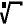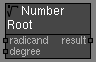# Number Root node

Left Toolbar >Math Nodes >Number Root

The Number Root node allows you to calculate an nth root of a Number value.

## Procedure

### To execute a root operation on two Node Values in the node graph:

1. On the left toolbar choose Math Nodes> Number Root2. Drag the Number Root icon into the Node Graph.3. Connect the Output: result.

## Inputs

radicand A NUMBER type of value.

degree A NUMBER type of value.

## Outputs

result The number value.

## Attributes PanelRadicand The first term in the root operation. This attribute is exposed as input by default.

Degree The second term in the root operation. This attribute is exposed as input by default.

Math Nodes

Main Page